# Python Program to Solve Quadratic Equation

In this tutorial you will learn about the Python Program to Solve Quadratic Equation and its application with practical example.

In this tutorial, we will learn to create a Python Program to Solve Quadratic Equation using python Programming.

## Prerequisites

Before starting with this tutorial we assume that you are best aware of the following Python programming topics:

• Python Operator.
• Basic Input and Output
• Basic Python  programming.
• Python if-else statements.
• Python Loop.

The Standard  Quadratic Equation looks like this: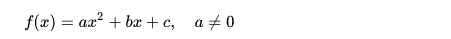Here,

• a, b and c are known values.and  ‘a‘ cannot be 0.
• x‘ is  unknown  variable.

The name Quadratic is come from Latin and means “to square”  from “quad” means square, because the variable gets squared (like x2).
Quadratic equations are second-degree algebraic expressions and are of form ax2 + bx + c = 0.

## Python Program to Solve Quadratic Equation

In this program we will create a program which will solve a Quadratic Equation.First we would declared and initialized the required variables. Next, we would prompt user to input the value. Later we will find solution of Quadratic Equation.

## Output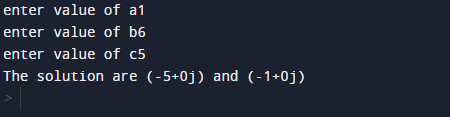In our program, we have first declared and initialized a set variables required in the program.

• a =taking value for a.
• b =taking value for b.
• c =taking value for c.
• s1=for solution one.
• s2=for solution  two

First of all  we declare variable and take three value from user and assign to ‘a’,’b’ and in ‘c’ variable.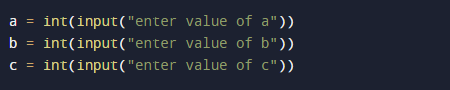After these values we will find  the value of discriminanthere we calculated the discriminant and then now we find the two possible solutions of the quadratic equation.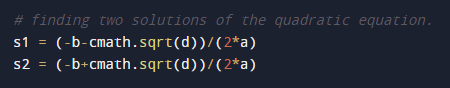In our program you can change coefficient and real numbers ‘a’,’b’and‘c’ and get different different solution of quadratic equation.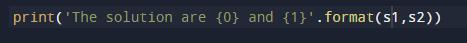In this tutorial we have learn about the Python Program to Solve Quadratic Equation and its application with practical example. I hope you will like this tutorial.